# Angular velocity using Newton's law

Huumah

## Homework Statement

Small object of mass m is placed on the inner surface of the conical dish which can rotate with angular speed ω.

For μ=0.3 calculate the minumum and the maximum ω such that the object is remains at rest. Solve the problem using the indicated axes x-y.

Do the following steps:
(a) Show all forces acting on the object and and the acceleration
(b) Write Newton’s second law along both axes and solve.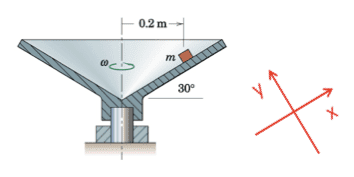F=ma=m*ω2*r

## The Attempt at a Solution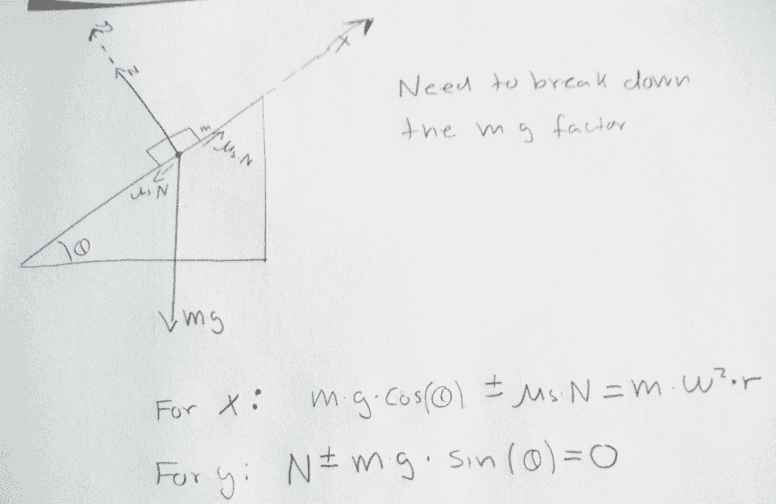I have plugged in numbers and got answers for ωmax and ωmin but I dont get the right answer.
I have solved it right when I choose axis the ordinary X-Y axis but I need to know how to solve with using the other axis mentioned in the problem

Last edited:

Saitama
Your equation for both the X and Y direction is wrong.
You have taken the wrong component of mg and mω2r along the X direction. In the RHS, it won't be completely mω2r, it will be some component of this force. Make a free body diagram.

Huumah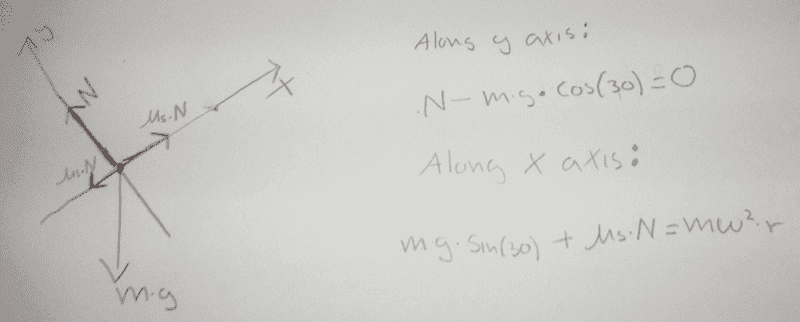In my free body diagram i have the fricton coefficent on both sides because it can go both upwards and downwards.

I dont really get what I should do on the right hand side for mω2*r

I have been trying for a few hours now and I am completely lost now.

Saitama
In my free body diagram i have the fricton coefficent on both sides because it can go both upwards and downwards.
Correct!
I dont really get what I should do on the right hand side for mω2*r
The force mω2r doesn't act in the X direction, so you will have to take its component along the X direction and solve.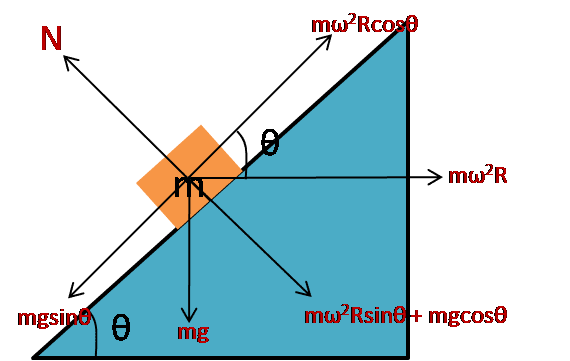Huumah
Thanks very much.

I did not know in what direction the ω2 force was heading. Tried to read it in my physics book but english is not my first language so i didn't understand it.

I feel like i'm getting closer but there is something wrong in my 2 equations because I cant solve them ( because there is something wrong with them)

Can you give my a hint, Sir?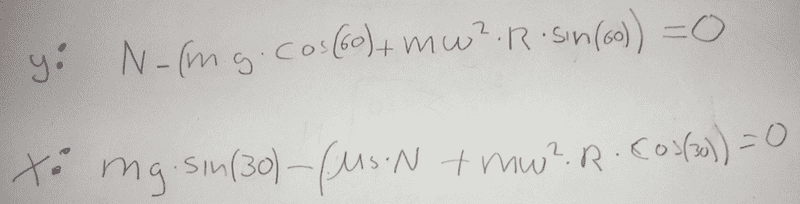Mentor
I did not know in what direction the ω2 force was heading. Tried to read it in my physics book but english is not my first language so i didn't understand it.
ω2r is the centripetal acceleration. Don't think of mω2r as a force, but as mass*acceleration. (View things from the usual inertial frame.)

I feel like i'm getting closer but there is something wrong in my 2 equations because I cant solve them ( because there is something wrong with them)
Why can't you solve them? Eliminate N. (I think you have one of your angles wrong.)

Huumah
I always got an error when i tried to solve it using wolfram alpha.

I just probably had a silly typo because it worked when I typed it in again.

I got it right when i changed the angle to 30° in the formula for y axis.

But I still dont get why it is not 60° in the y axis formula.

Anyway thank you both so much for the help. Such a lovely community.

Mentor
I got it right when i changed the angle to 30° in the formula for y axis.

But I still dont get why it is not 60° in the y axis formula.
The angle between mg and your y axis is 30 degrees; the angle between mω2r and your y axis is 60 degrees. And sin(30)=cos(60) & sin(60)=cos(30).